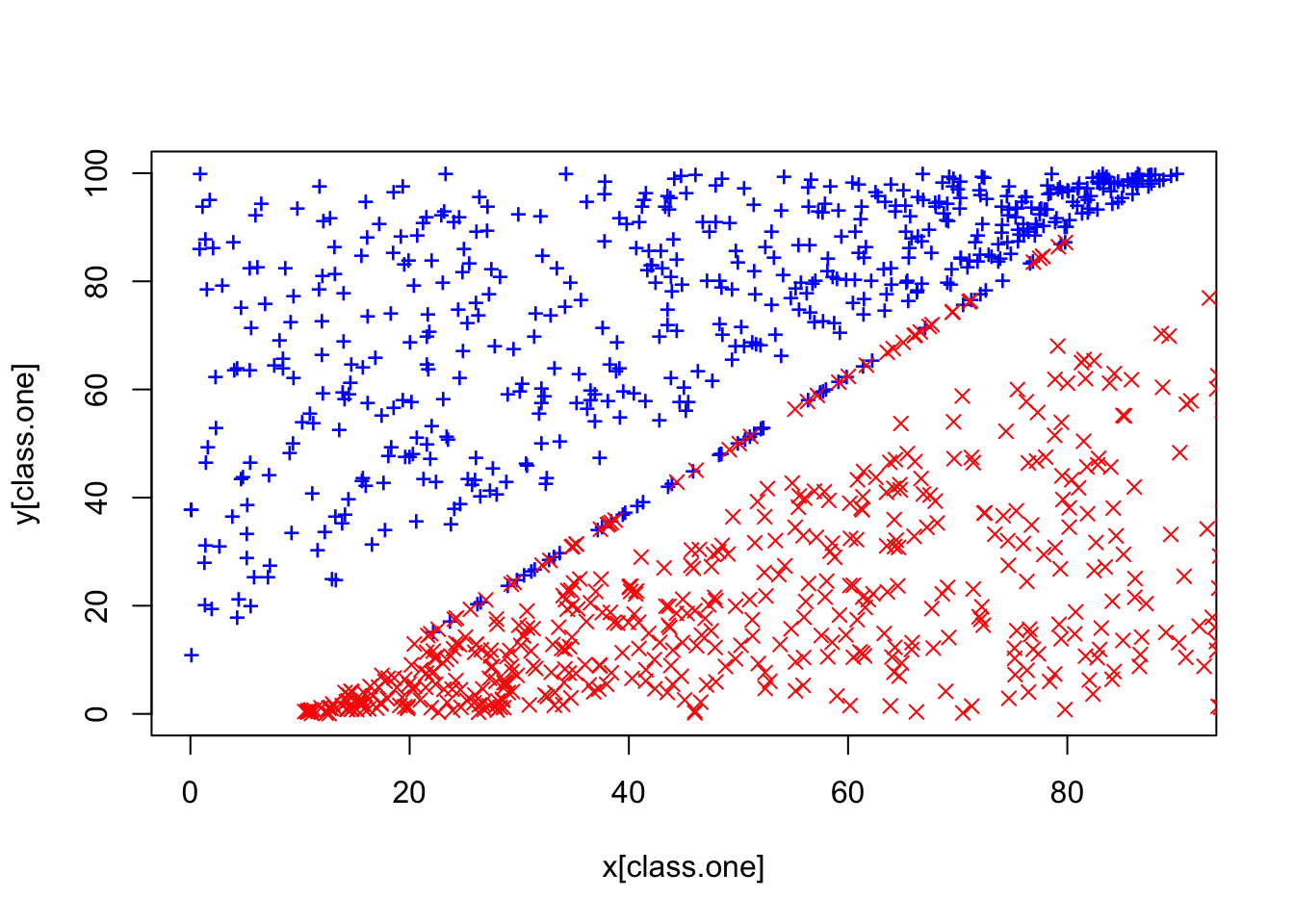ISLR Home

# Question

p370

At the end of Section 9.6.1 (the Lab), it is claimed that in the case of data that is just barely linearly separable, a support vector classifier with a small value of cost that misclassifies a couple of training observations may perform better on test data than one with a huge value of cost that does not misclassify any training observations. You will now investigate this claim.

1. Generate two-class data with p = 2 in such a way that the classes are just barely linearly separable.

2. Compute the cross-validation error rates for support vector classifiers with a range of cost values. How many training errors are misclassified for each value of cost considered, and how does this relate to the cross-validation errors obtained?

3. Generate an appropriate test data set, and compute the test errors corresponding to each of the values of cost considered. Which value of cost leads to the fewest test errors, and how does this compare to the values of cost that yield the fewest training errors and the fewest cross-validation errors?

# 6a Generate Data

Generate two-class data with p = 2 in such a way that the classes are just barely linearly separable.

From the lab. Taking Prince’s data generation. FIXME!!

``````set.seed(3154)
# Class one
x.one = runif(500, 0, 90)
y.one = runif(500, x.one + 10, 100)
x.one.noise = runif(50, 20, 80)
y.one.noise = 5/4 * (x.one.noise - 10) + 0.1

# Class zero
x.zero = runif(500, 10, 100)
y.zero = runif(500, 0, x.zero - 10)
x.zero.noise = runif(50, 20, 80)
y.zero.noise = 5/4 * (x.zero.noise - 10) - 0.1``````

## Combine all

``````class.one = seq(1, 550)
x = c(x.one, x.one.noise, x.zero, x.zero.noise)
y = c(y.one, y.one.noise, y.zero, y.zero.noise)

{plot(x[class.one], y[class.one], col = "blue", pch = "+", ylim = c(0, 100))
points(x[-class.one], y[-class.one], col = "red", pch = 4)
}````````````df = data.frame(x=x[class.one], y = y[class.one])
df2 = data.frame(x=x[-class.one], y = y[-class.one])

p <- ggplot(df, aes(x=x, y=y, color='black', size=4))``````
• positive/blue x negative/red
``````p + geom_point(color='blue', size=3, shape="+") +
geom_point(data=df2, color='red', size=4, alpha = 1/2, shape=4) + xlim(0, 100)``````